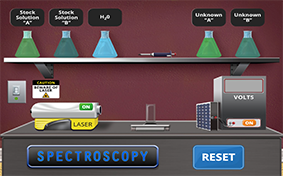# SpectroscopyImagine that you are in charge of a police crime laboratory. You receive a sample and need to know how much of a solute is dissolved in the sample. Your challenge is to determine the concentration of an unknown sample given a set of standard solutions. Are you ready to begin? You will have to "concentrate" to solve this crime.

### What is the difference between qualitative and quantitative analysis?

Qualitative analysis focuses on description, while quantitative analysis focuses on numerical values. For example, if you were observing a piece of clay, qualitative analysis would be determining what shape the clay is, its color, and what type of clay it is. Quantitative analysis would be determining the mass of the clay, its dimensions, and its density.

### What is the difference between a dilute and concentrated solution?

A diluted solution is one in which there is not very much solute compared to the amount of solvent. A concentrated solution is one in which there is a large amount of solute compared to the amount of solvent. For example, if you were mixing sugar (the solute) into water (the solvent), if you only put a little bit of sugar into the water the solution would be a dilute, but if you put a lot of sugar into the water, the solution would be concentrated.

### What is a spectroscope and how does it work?

A spectroscope breaks light down into its different wavelengths. Light acts as both a particle and a wave, and has properties of both. Therefore, light acts in a similar manner as ocean waves, including having a wavelength. Different colors of light have different wavelengths. Red light has the longest wavelength, and violet light has the shortest. When objects are heated up, they emit light that is made up of specific wavelengths. A spectroscope can determine what wavelengths make up the light that is emitted, and from this data the type of substance can be determined. Light enters the spectroscope through a tube that has a small slit and lens in it. The slit and lens make the light rays that are entering the spectroscope parallel to each other. The light then passes through a prism, which diffracts the light and separates it into bands of each wavelength that make up the light. There is a small telescope on the spectroscope that allows the user to see the bands and determine what their wavelength is.

### What is the Beer-Lambert Law?

Beer-Lambert Law is named for the scientists who developed the idea, August Beer and Johann Lambert. The law discusses the relationship between the concentration of a solution and how much light it absorbs. It states that A = -log (I/I0), where A is the absorbance of the light by the solution, I0 is the intensity of the light before it goes through the solution, and I is the intensity of the light after it goes through the solution. According to the law, a more concentrated solution will absorb more light than a more diluted solution.

### What is a logarithm?

A logarithm is how many times a certain number must be multiplied by itself to get another number. For example, if you want to know how many 2's you need to multiply to get 8, the logarithm is 3, because 2 × 2 × 2 = 8. To express this mathematically, you would write log2 8 = 3, read "log base 2 of 8 is 3", which simply means that you need to multiply 2 by itself 3 times to get 8. Another way of thinking of this is that a logarithm is the power that you need to raise the base to in order to get another number. Using the same example, the base is 2, and the logarithm is 3, because 23 = 8.